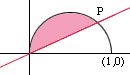There is a line through the origin that divides the region bounded by the parabola y=x-x2 and the x-axis into two regions with equal area. What is the slope of the line? Could you please solve the problem in detail and show me step by step how to do it. My name is Kerry. I am a student in calculus in the 12th grade. thank you Kerry Hi Kerry, I drew a rough sketch of the parabola and the line y = m x that divides the region into two pieces of equal areas.I would first find the area between the parabola and the x-axis. I got 1/6. Thus the area of the region below the parabola and above the line y = m x, the region shaded pink in the diagram, must be one-half of 1/6, that is 1/12. To calculate the area of this region you need to know the X-coordinate of P, the point where the parabola intersects the line. Solving the equation of the parabola with y = m x gives an X-coordinate of 1 - m. Finally use calculus to find the area of the region below the parabola and above the line, set this value equal to 1/12 and solve for m. Cheers, Penny Go to Math Central